# Projective straight line

(diff) ← Older revision | Latest revision (diff) | Newer revision → (diff)

projective line

A projective space of dimension. A projective line, considered as an independent object, is a closed one-dimensional manifold. A projective line is a special (and peculiar) projective space: there are no interesting incidence relations on it similar to those on projective spaces of higher dimensions. The only invariant of a projective line is the number of its points. A projective line is called continuous, discrete or finite if it is incident to a point set of the cardinality of the continuum, of countable cardinality or of finite cardinality, respectively.

A projective line is called ordered if a relation of separation of two pairs of distinct points is given on it. It is assumed that this relation does not depend on the order of the pairs or on the order of the points in the pairs, and that any quadruple of distinct points splits uniquely into two mutually separating pairs. In addition, the location axiom is adopted, which connects five distinct points (see, for example, ). The ordering of a projective line over the fieldis related to the ordering of the field. Namely, a pair of points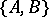separates a pair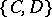if the cross ratio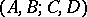is negative, and does not separate ifis positive. The finite projective line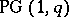over a Galois field of odd ordercan be ordered similarly to the real projective line. It is assumed (see ) that a pairof points separates a pairif and only ifis a quadratic residue in the Galois field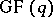.

A projective line acquires a certain geometric structure if it is imbedded in a projective space of higher dimension. For example, a projective line is uniquely determined by two distinct points, while the analytic definition of a projective line as a set of equivalence classes of pairs of elements of a skew-fieldthat are not simultaneously equal to zero, is actually equivalent to an imbedding of the projective line into a projective space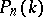,. If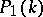is the projective line over the field, then the group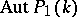of automorphisms of the projective line can be represented on the points ofin parametric form as the set of mappings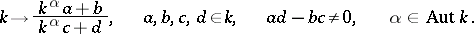The group of algebraic automorphisms of the real projective line is isomorphic to the group of displacements of the real hyperbolic plane, and the order of the groupequals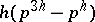.

On a projective line one can construct different geometries. For example, the Möbius plane of orderadmits an interpretation on the projective line(see ). Another traditional geometric construction is the representation of the projective spaceon the projective line(see ) under which a point ofis represented by an-tuple of points of the projective line(hereis an algebraically closed field).

How to Cite This Entry:
Projective straight line. Encyclopedia of Mathematics. URL: http://encyclopediaofmath.org/index.php?title=Projective_straight_line&oldid=17117
This article was adapted from an original article by V.V. Afanas'ev (originator), which appeared in Encyclopedia of Mathematics - ISBN 1402006098. See original article